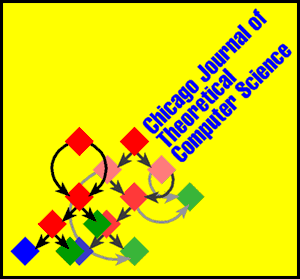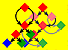# Pictorial Logo for the Chicago Journal of Theoretical Computer ScienceCreated by Chad Kainz of the Multimedia and Visualization Center at the University of Chicago.

## Picture of an Infinite Computation

The pictorial logo for the Chicago Journal of Theoretical Computer Science represents the combinatory expression SII(SII) (left foreground) reducing to I(SII)(I(SII)) (right background). In two more steps, the expression reduces further to SII(I(SII)) and SII(SII), which is the same as the starting expression, showing that reduction will go on infinitely. In the pictorial version, red squares represent the binary operation of application, blue squares represent the symbol S, and green squares represent the symbol I. Arrows link application squares to a function (left arrow) and its argument (right arrow). The repeated subexpression SII is shared, rather than represented twice.

## Combinatory Calculus as a Very Small Programming Language

Theoretical computer science, which studies the abstract mathematical nature of computation, has provided several key insights into the structural properties that must hold in all computing systems, no matter how they are designed and implemented. Several of these insights reveal inherent and unavoidable limitations on computing systems. Desirable properties of a computing system may ineluctably entail other, undesirable properties. For example, in order to be completely general-purpose, that is, to have the desirable capability of computing all of the functions that are in principle computable, a computing system must also provide programs that compute forever uselessly, producing no output. In fact, all programming languages in widespread use today have chosen generality, at the cost of making each programmer face the risk of accidentally producing an infinite computation.

Theoretical computer science has also clarified the programming resources that are required in order to provide the power to compute all computable functions. They are amazingly simple. One of the simplest systems that is powerful enough for general-purpose computing is the Combinator Calculus, which is carried out in a language involving only one binary operator and two constants.

The binary operator of the Combinator Calculus is called "application," intended to capture the intuitive idea of applying a function to an argument, or a program to an input. An expression of the form MN represents the application of the subexpression M (function or program) to the subexpression N (argument or input). Every expression in the language of combinators is allowed to serve as a function/program and also as an argument/input (this is sensible since programs are written out as special sorts of texts, and those texts are easily read as inputs by programs). The application operation itself does not require a symbol: it is represented by the juxtaposition of its operands, just as multiplication is represented by juxtaposition in conventional numerical mathematics. Unlike multiplication, application is not associative. That is, (MN)P is not always equal to M(NP). To avoid proliferation of parentheses, we agree to associate applications to the left whenever possible. So, MNPQ is read as ((MN)P)Q. The alternative (MN)(PQ) can be written MN(PQ), but the rightmost parentheses cannot be omitted.

The two constants required by the Combinator Calculus are written S and K. The language of the Combinator Calculus contains precisely those expressions that can be written by combining Ss and Ks, using the binary application operation. For example, K(SK) and S(KS)(SK) are expressions in the language of the Combinator Calculus. There are no variables or other sorts of symbols or operations besides the constants S and K and the implicit application operation.

Finally, the Combinator Calculus has two rules of calculation, one for each of its constants:

• SMNP reduces to MP(NP)
• KMN reduces to M
Calculation in the Combinator Calculus is called "reduction." When calculating from an expression Q, we are allowed to replace any subexpression of Q that has the form SMNP or the form KMN by the corresponding reduced form MP(NP) or M, respectively. Notice that we have used M, N, and P as variables standing for arbitrary expressions in order to describe the rules of calculation, but no variables are required in specific calculations. The symbol K can be understood as a constructor of constant functions (K stands for "konstant" in German). An expression of the form KM represents the constant M function, since whatever it is applied to, the result of reduction is M. The symbol S is harder to understand intuitively. I think of it as a kind of copying "shuffle." SMN represents a function that copies its argument, feeding one copy as input to M, the other to N, and then applies the two results together.

Perhaps S and K appear rather weak at first, but together they are capable of computing every function that is in principle computable. For a very simple example, notice that the identity function may be defined as SKK. That is, for every expression M, SKKM reduces to KM(KM), which reduces to M. The identity function is so important that it is often given its own symbol, I, and the rule that IM reduces to M. In the infinite loop in the logo, I have used the third constant I for brevity, but it may be replaced everywhere by SKK and the same computation works, with a few more steps.

Whether we regard I as a third constant, or merely as an abbreviation for SKK, the expression SII(SII) is important, because it yields the simplest example of an infinite computation in the Combinator Calculus. The mere presence of infinite computation does not prove that the Combinator Calculus can define all of the computable functions, but it is one crucial requirement for that generality. Notice that SII(SII) reduces to I(SII)(I(SII)) (by the S rule with M=I, N=I, and P=SII), then to SII(I(SII)), and then to SII(SII), and so on ad infinitum. The first step in that infinite reduction is shown by the logo. SII may be understood as an operator that takes one argument and applies that argument to itself. So, SII(SII) is self-application self-applied.

SII(SII) is called the "paradoxical combinator," because it demonstrates the same self-applicative schema that drives Russell's "paradox" (more properly, it is an antinomy). Bertrand Russell defined the set of all sets that do not contain themselves and asked whether that set contains itself. Asking whether a set contains itself has the same formal structure as applying a function to itself. Haskell B. Curry took advantage of the particular simplicity of SII(SII) to construct another antinomy (also popularly called "Curry's paradox"), involving only implication and self-reference, without sets or negation. Curry defined the moon-cheese proposition that says, "if this proposition is true, then the moon is made of cheese."

• Assume that the moon-cheese proposition is true.
• It follows by the implication in this proposition that the moon is made of cheese.
• So, the truth of the moon-cheese proposition implies that the moon is made of cheese.
• But, such an implication is precisely what the moon-cheese proposition asserts, so the moon-cheese proposition is true.
• So, the moon is made of cheese.
Clearly, any ridiculous claim can be substituted for "the moon is made of cheese," so the fault is in the logical form of the argument, rather than in our data regarding the moon. That fault is remarkably difficult to describe, since each individual step of reasoning looks safe on the surface.

A lot more work is required to demonstrate that the Combinator Calculus can define all of the computable functions. One key element is the encoding of the integers 0, 1, 2, 3, etc. by the expressions KI, S(S(KS)K)(KI), S(S(KS)K)(S(S(KS)K)(KI)), etc. The expression KI represents 0, and S(S(KS)K) represents the incrementation function that adds 1 to its argument. The expression representing the integer i may also be understood as a function which, taking another function f as its argument, composes f with itself i times.

Another key element in general-purpose computing is a mechanism for performing recursive computation. Given an expression M to be applied recursively, construct S(KM)(SII)(S(KM)(SII)). This expression reduces in the sequence

• S(KM)(SII)(S(KM)(SII))
• KM(S(KM)(SII))(SII(S(KM)(SII)))
• M(SII(S(KM)(SII)))
• M(I(S(KM)(SII))(I(S(KM)(SII))))
• M(S(KM)(SII)(I(S(KM)(SII))))
• M(S(KM)(SII)(S(KM)(SII)))
Notice that the subexpression following the initial occurrence of M is exactly the same as the starting expression. So, further reduction will produce
• M(M(S(KM)(SII)(S(KM)(SII))))
• M(M(M(S(KM)(SII)(S(KM)(SII)))))
and so on ad infinitum. Notice that the self-application operator SII from the paradoxical combinator is involved in useful recursions, as well as in the silly repetitive computation of the paradoxical combinator.

The reduction sequence above gives the essential ideas in a proof of the famous and crucial recursion theorem. A pictorial version of that proof could be very elegant, but a bit too big for a logo. In principle, a whole operating system could be written as a combinatory expression. Pictorial versions of the workings of that operating system could provide murals for all the buildings in the world.

## History of the Combinator Calculus

The idea of the combinators was invented by Schönfinkel (1924), and independently by Haskell B. Curry (1930), who systematized the study of the "theory of combinators." Schönfinkel and Curry were largely concerned with demonstrating that the formal manipulations of mathematical logic could be reduced to a very primitive set of finitary operations, without depending on relatively complex and global notions such as substitution for free occurrences of variables. Curry, in particular, connected the theory of combinators to logic in deep ways, through the formulae-as-types idea (due also to William Howard), and through his formalist philosophy of mathematics. In some sense, the combinatory calculus behaves as a machine language for mathematical logic, into which more sophisticated systems may be compiled. Interestingly, the Combinator Calculus also provides a natural sort of abstract machine language underlying functional programming. David A. Turner (1979) pointed out the practical value of this view of the Combinator Calculus. Many important techniques in compiling functional programming languages are based on the Combinator Calculus and variants of it using different sets of constants.

## Bibliography

1. Haskell B. Curry. Grundlagen der Kombinatorischen Logik. American Journal of Mathematics, 52 (1930), pp. 509-536 and 789-834.
2. Haskell B. Curry and R. Feys. Combinatory Logic, Volume I. North-Holland, 1958.
3. J. Roger Hindley and Jonathan P. Seldin. Introduction to Combinators and Lambda Calculus. London Mathematical Society Student Texts 1, Cambridge University Press, 1986.
4. M. Schönfinkel. Über die Bausteine der mathematischen Logik. Mathematische Annalen 92 (1924), pp. 305-316. English translation in From Frege to Gödel, Jan van Heijenoort editor, Harvard University Press, 1967, pp. 355-366.
5. Smullyan, Raymond Merrill. To Mock a Mockingbird: and other logic puzzles including an amazing adventure in combinatory logic. Oxford University Press, 1990. Originally published by Knopf, New York, 1985.
6. Sören Stenlund. Combinators, Lambda Terms, and Proof Theory. Synthese Library, D. Reidel, 1972.
7. David A. Turner. A new implementation technique for applicative languages. Software - Practice and Experience, 9 (1979), pp. 31-49.

## Exportable Versions of the Logo and Related Graphics

• Picture plus title, square
• 107 by 100 pixels GIF
• 150 by 140 pixels GIF
• Picture plus title, horizontal
• 116 by 50 pixels GIF
• 232 by 100 pixels GIF
• 357 by 154 pixels GIF (top of most journal pages)
• Picture only
• 68 by 50 pixels GIF (the button)
• 135 by 100 pixels GIF
• 208 by 154 pixels GIF
• 28 by 13 pixels, partial picture GIF (fits inline with text)
• Title only
• 48 by 50 pixels GIF
• 97 by 100 pixels GIF
• 157 by 162 pixels GIF
• Color-co-ordinated arrows, all 16 by 16 pixel GIFs
• UP
• DOWN
• RIGHT
• LEFT

•Michael J. O'Donnell <odonnell@cs.uchicago.edu>